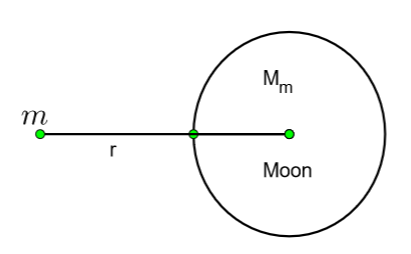QuestionAnswers

# If ${{\text{M}}_{\text{e}}}$ is the mass of earth and ${{\text{M}}_{\text{m}}}$ is the mass of moon $\left( {{\text{M}}_{\text{e}}}\text{=81}{{\text{M}}_{\text{m}}} \right)$ . The potential energy associated with object of mass m situated at a distance R from the centre of earth and r from the centre of moon will be:\begin{align} & \text{A}\text{.-Gm}{{\text{M}}_{\text{m}}}\left[ \dfrac{\text{R}}{\text{81}}\text{+r} \right]\dfrac{\text{1}}{{{\text{R}}^{\text{2}}}} \\ & \text{B}\text{.-Gm}{{\text{M}}_{\text{m}}}\left[ \dfrac{\text{81}}{\text{r}}\text{+}\dfrac{\text{1}}{\text{R}} \right] \\ & \text{C}\text{.-Gm}{{\text{M}}_{\text{m}}}\left[ \dfrac{\text{81}}{\text{R}}\text{+}\dfrac{\text{1}}{\text{r}} \right] \\ & \text{D}\text{.-Gm}{{\text{M}}_{\text{m}}}\left[ \dfrac{\text{81}}{\text{R}}\text{-}\dfrac{\text{1}}{\text{r}} \right] \\ \end{align}Verified
128.7k+ views
Hint: Gravitational potential energy is the energy that an object acquires when there is a change in its position in a gravitational field. It is equivalent to the work done against gravity to bring a mass to a given point in space.
Formula used:
Gravitational potential energy is given by the equation
$\text{U=}\dfrac{\text{-GMm}}{\text{r}}$ , where G is gravitational constant, M is mass of the attracting body, m is the mass of the body being attracted, r is the distance between their centers.

We are given that ${{\text{M}}_{\text{e}}}$ is the mass of earth and ${{\text{M}}_{\text{m}}}$ is the mass of the moon. Relation between mass of earth and mass of moon is given to us, ${{\text{M}}_{\text{e}}}\text{=81}{{\text{M}}_{\text{m}}}$
We are asked to find the potential energy of a body of mass ‘m’. This body is situated at a position of distance R from the centre of earth and distance r from the centre of moon.
Now let us consider the situation from earth.A body of mass ‘m’ is situated at a distance of R from the centre of the earth.
Potential energy exerted on the body by the earth is given by,
${{\text{U}}_{\text{e}}}\text{=}\dfrac{\text{-Gm}{{\text{M}}_{\text{e}}}}{\text{R}}$ , were ‘G’ is gravitational constant, ‘m’ is mass of the body, ‘${{\text{M}}_{\text{e}}}$’ is mass of earth and R’’ is the distance from centre of earth to the body.
Now let us consider the moon

Potential energy exerted on the body by moon is given by
${{\text{U}}_{\text{m}}}\text{=}\dfrac{\text{-Gm}{{\text{M}}_{\text{m}}}}{\text{r}}$ , were ‘G’ is gravitational constant, ‘m’ is mass of the body, ‘${{\text{M}}_{\text{m}}}$’ is mass of moon and ‘r’ is the distance from centre of moon to the body.

Total potential energy experienced by the body will become,
$\text{U=}{{\text{U}}_{\text{e}}}\text{+}{{\text{U}}_{\text{m}}}$
$\text{U=}\dfrac{\text{-Gm}{{\text{M}}_{\text{e}}}}{\text{R}}\text{+}\dfrac{\text{-Gm}{{\text{M}}_{\text{m}}}}{\text{r}}$

It is given that ${{\text{M}}_{\text{e}}}$​=81${{\text{M}}_{\text{m}}}$​. Hence we can rewrite the above equation.
$\text{U=}\dfrac{\text{-Gm }\!\!\times\!\!\text{ 81 }\!\!\times\!\!\text{ }{{\text{M}}_{\text{m}}}}{\text{R}}\text{+}\dfrac{\text{-Gm}{{\text{M}}_{\text{m}}}}{\text{r}}$
Common terms in this equation can be taken outside and equation can be rewritten as
$\text{U=-Gm}{{\text{M}}_{\text{m}}}\left[ \dfrac{\text{81}}{\text{R}}\text{+}\dfrac{\text{1}}{\text{r}} \right]$

This is the potential energy experienced by the body due to the moon and earth.
So, the correct answer is “Option C”.

Note:
From the work done against the force of gravity to bring a mass in form infinity where the potential energy is given a value of zero, the term for gravitational potential energy can be written as
$\text{U=}\dfrac{\text{-GMm}}{\text{r}}$, where ‘G’ is gravitational constant, ‘M’ is mass of the attracting body, ‘m’ is the mass of the body being attracted, ‘r’ is the distance between their centers.
However, the acceleration of gravity g can be considered approximately constant for objects near the earth, and the expression of potential energy relative to the earth's surface becomes
$\text{U=mgh}$ , where ‘m’ is mass of the body, ‘g’ surface value of acceleration due to gravity and ‘h’ is height above the surface.